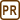## Total change of entropy in Carnot cycle

### Task number: 2117

Prove that the total change of entropy in the Carnot Cycle is zero.

• #### Help 1 - Carnot cycle

Remember, what processes the Carnot Cycle consists of. Specify the change of entropy for each of these processes.

• #### Hint 2 - Change of entropy in adiabatic processes

Entropy does not change during adiabatic processes, because dQ = 0.

• #### Hint 3 - The change of entropy in isothermal process

The gas does work W during the isothermal process and it absorbs heat Q, increasing its entropy by

$\Delta S=\frac{Q}{T},$

where T is the temperature at which the process takes place.

The heat Q is equal to the work W, which we calculate by using the integral calculus.

During isothermal expansion, the gas is working at the expense of the heat absorbed, hence the entropy is growing. In isothermal compression, the work done by the gas is negative (the surroundings do work), i.e. the heat absorbed is also negative (the gas gives out heat to the surroundings). The change of entropy is also negative, i.e. entropy is decreasing.

• #### Hint 4 - Expressing the pressure

Use the so-called Boyle-Mariotte law to express the pressure p as a function of the volume V.

• #### Analysis

The Carnot Cycle is a thermodynamic cycle that consists of four successive reversible processes: isothermal expansion, adiabatic expansion, isothermal compression, and adiabatic compression.

The change in entropy is equal to the ratio of the heat absorbed and the temperature at which the heat was absorbed.

Entropy does not change during adiabatic processes. It is due to the fact that there is no heat exchange between the gas and its surroundings.

During the isothermal expansion, the gas does work and it accepts heat from the surroundings; therefore its entropy increases by the amount given by the ratio of the absorbed heat and the thermodynamic temperature. The absorbed heat is equal to the work that gas does in this process. To calculate this work, we must use the integral calculus because the pressure is not constant in this process. In order to express the pressure as a function of the volume, we use the so-called Boyle-Mariotte law.

During the isothermal compression, work is done by the surroundings and the gas gives out heat; therefore its entropy decreases by the amount given by the ratio of the given heat and the thermodynamic temperature. The heat that left the system is equal to the work done by the surroundings. We must again use the integral calculus to compute it.

Our task is to show that both these changes of entropy are of same size.

• #### Solution

The Carnot Cycle is a thermodynamic cycle that consists of four reversible processes: isothermal expansion, adiabatic expansion, isothermal compression, and adiabatic compression.

We will now determine the entropy changes in each phase of the cycle.

Entropy does not change during the course of both adiabatic processes. This is due to the fact that there is no heat exchange between the gas and its surroundings. It holds dQ = 0, and thence also

$\text{d}S=\frac{\text{d}Q}{T}=0,$

where T is the thermodynamic temperature.

We get the following change of entropy in our case

$\Delta S_2=\Delta S_4=0.$

During isothermal expansion, the gas does work, it absorbs heat Q1, and its entropy increases by

$\Delta S_1=\frac{Q_1}{T_2},$

where T2 is the temperature at which the process takes place.

The absorbed heat Q1 is equal to the work W1 that the gas does during this process. We need to use the integral calculus to calculate it

$Q_1=W_1= \int\limits_{V_1}^{V_2}p\,\text{d}V,$

because the pressure p is not constant. We use initial and final volumes of the gas V1 and V2 as the limits of the integral.

We express pressure p as a function of the volume V using the so-called Boyle-Mariotte law p1V1 = pV. We put the obtained expression

$p=\frac{p_1V_1}{V}$

into the integral

$W_1= \int\limits_{V_1}^{V_2}\frac{p_1V_1}{V}\text{d}V=$

we factor out the constants in front of the integral

$=p_1V_1\int\limits_{V_1}^{V_2}\frac{\text{d}V}{V}=$

we calculate the integrate and evaluate it at the limits

$=p_1V_1[\ln{V}]_{V_1}^{V_2}=p_1V_1\ln{\frac{V_2}{V_1}}.$

After using the ideal gas law p1V1 = nRT2, we get

$W_1= nRT_2\ln{\frac{V_2}{V_1}}.$

We then get for ΔS1

$\Delta S_1=\frac{Q_1}{T_2}= nR\ln{\frac{V_2}{V_1}}.$

Now we have to determine the change of entropy ΔS3 during the isothermal compression. In this case, the surroundings do work, the gas emits heat, and the entropy decreases therefore. In the concrete, it decreases by the value

$\Delta S_3=\frac{Q_3}{T_1},$

where T1 is the temperature at which the process takes place.

The heat Q3 is equal to the work W3 done by the surroundings. To calculate it again, we must use the integral calculus. The procedure is similar as with the previous process

$Q_3=W_3=-\int\limits_{V_3}^{V_4}p\,\text{d}V= -p_3V_3\int\limits_{V_3}^{V_4}\frac{\text{d}V}{V}=$ $=p_3V_3\ln{\frac{V_3}{V_4}}= nRT_1\ln{\frac{V_3}{V_4}}.$

We then get the following change of entropy ΔS3

$\Delta S_3=\frac{Q_3}{T_1}= nR\ln{\frac{V_3}{V_4}}.$

The total change in entropy is, based on the considerations above, given by the equation

$\Delta S=\Delta S_1-\Delta S_3= nR\left(\ln{\frac{V_2}{V_1}}-\ln{\frac{V_3}{V_4}}\right).$

For the Carnot Cycle, one can prove (see Comment) the validity of the relationship

$\frac{V_2}{V_1}=\frac{V_3}{V_4}.$

After using this equality we get

$\Delta S= nR\left(\ln{\frac{V_2}{V_1}}-\ln{\frac{V_2}{V_1}}\right)= 0.$

The proof is thus complete.

• #### Answer

We proved that the total change of entropy during the Carnot Cycle is zero.

• #### Comment

Let us derive in addition the above used formula:

$\frac{V_2}{V_1}=\frac{V_3}{V_4}.$

We start from the ideal gas law for adiabatic processes in the form

$TV^{\kappa-1}=\mathrm{konst.},$

where κ is the Poisson constant (the heat capacity ratio).

In the case of adiabatic expansion, it holds:

$T_1V_2^{\kappa-1}=T_2V_3^{\kappa-1}.$

For the adiabatic compression we get:

$T_2V_4^{\kappa-1}=T_1V_1^{\kappa-1}$

or the other way around:

$T_1V_1^{\kappa-1}=T_2V_4^{\kappa-1}.$

We divide the last form of compression equation with the expansion equation and we get the formula:

$\left(\frac{V_2}{V_1}\right)^{\kappa -1}=\left(\frac{V_3}{V_4}\right)^{\kappa -1}.$

And taking the (κ−1)-th root, we get

$\frac{V_2}{V_1}=\frac{V_3}{V_4}.$